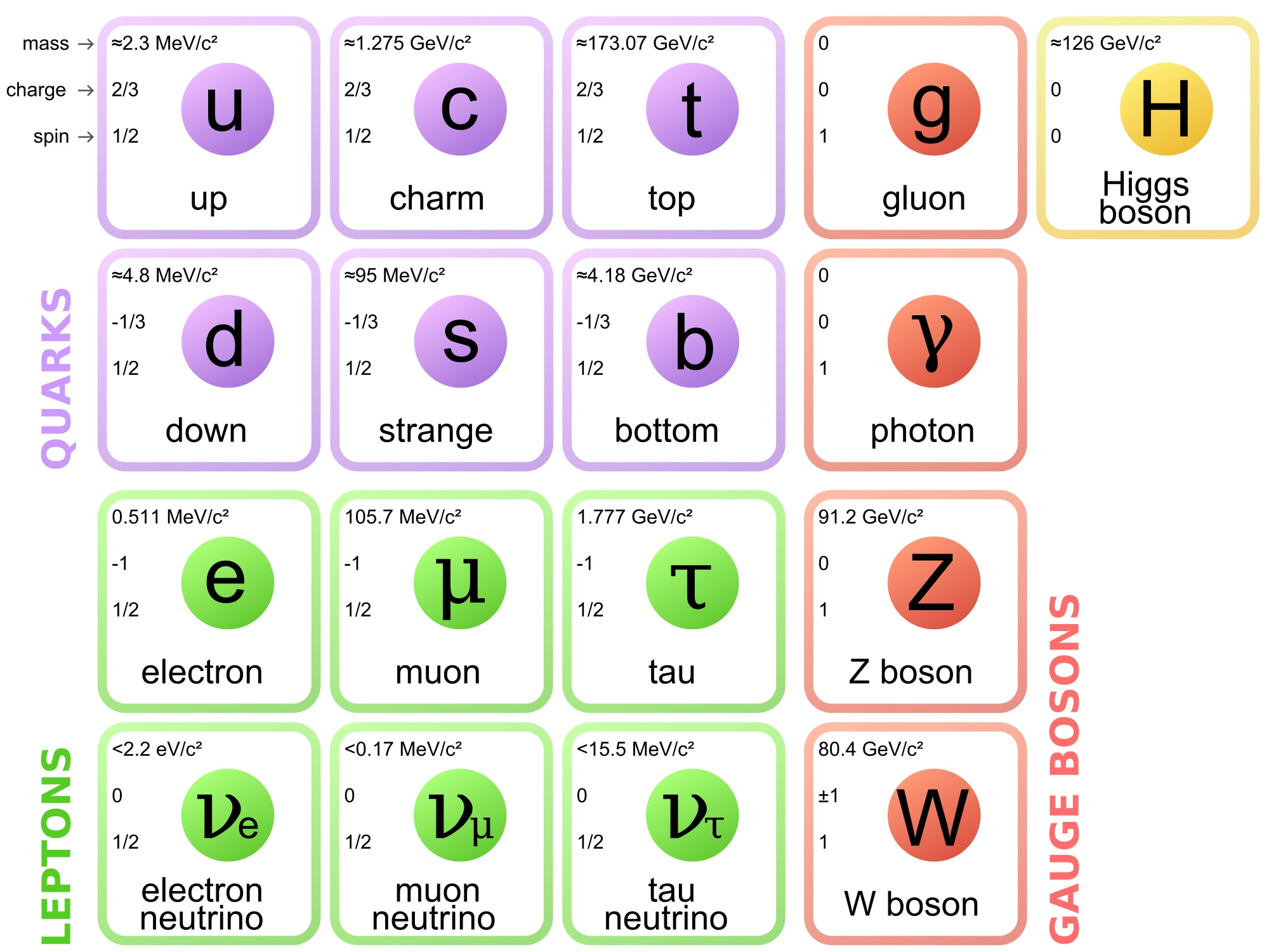## From Quantum Diaries: “The Standard Model: a beautiful but flawed theory”March 14th, 2014

This is the first part of a series of three on supersymmetry, the theory many believe could go beyond the Standard Model. First I explain what is the Standard Model and show its limitations. Then I introduce supersymmetry and explain how it would fix the main flaws of the Standard Model. Finally, I will review how experimental physicists are trying to discover “superparticles” at the Large Hadron Collider at CERN.

The Standard Model describes what matter is made of and how it holds together. It rests on two basic ideas: all matter is made of particles, and these particles interact with each other by exchanging other particles associated with the fundamental forces.

The basic grains of matter are fermions and the force carriers are bosons. The names of these two classes refer to their spin – or angular momentum. Fermions have half-integer values of spin whereas bosons have integer values as shown in the diagram below.The Standard Model of elementary particles, with the three generations of matter, gauge bosons in the fourth column, and the Higgs boson in the fifth.

Fermions come in two families. The leptons family has six members, with the electron being the best known of them. The quarks family contains six quarks. The up and down quarks are found inside protons and neutrons. The twelve fermions are the building blocks of matter and each one has a spin value of ½.

These particles interact with each other through fundamental forces. Each force comes with one or more force carriers. The nuclear force comes with the gluon and binds the quarks within the proton and neutrons. The photon is associated with the electromagnetic force. The weak interaction is responsible for radioactivity. It comes with the Z and W bosons. All have a spin of 1.

The main point is: there are grains of matter, the fermions with spin ½, and force carriers, the bosons with integer values of spin.

The Standard Model is both remarkably simple and very powerful. There are complex equations expressing all this in a mathematical way. These equations allow theorists to make very precise predictions. Nearly every quantity that has been measured in particle physics laboratories over the past five decades falls right on the predicted value, within experimental error margins.

So what’s wrong with the Standard Model? Essentially, one could say that the whole model lacks robustness at higher energy. As long as we observe various phenomena at low energy, as we have done so far, things behave properly. But as [particle] accelerators are getting more and more powerful, we are about to reach a level of energy which existed only shortly after the Big Bang where the equations of the Standard Model start getting shaky.

This is a bit like with the laws of physics at low and high speed. A particle moving at near the speed of light cannot be described with the simple laws of mechanics derived by [Isaac] Newton. One needs special relativity to describe its motion.

One major problem of the Standard Model is that it does not include gravity, one of the four fundamental forces. The model also fails to explain why gravity is so much weaker than the electromagnetic or nuclear forces. For example, a simple fridge magnet can counteract the gravitational attraction of a whole planet on a small object.

This huge difference in the strength of fundamental forces is one aspect of the “hierarchy problem”. It also refers to the wide range in mass for the elementary particles. In the table shown above, we see the electron is about 200 times lighter than the muon and 3500 times lighter than the tau. Same thing for the quarks: the top quark is 75 000 times heavier than the up quark. Why is there such a wide spectrum of masses among the building blocks of matter? Imagine having a Lego set containing bricks as disparate in size as that!

The hierarchy problem is also related to the Higgs boson mass. The equations of the Standard Model establish relations between the fundamental particles. For example, in the equations, the Higgs boson has a basic mass to which theorists add a correction for each particle that interact with it. The heavier the particle, the larger the correction. The top quark being the heaviest particle, it adds such a large correction to the theoretical Higgs boson mass that theorists wonder how the measured Higgs boson mass can be as small as it was found.

This seems to indicate that other yet undiscovered particles exist and change the picture. In that case, the corrections to the Higgs mass from the top quark could be cancelled out by some other hypothetical particle and lead to the observed low Higgs boson mass. Supersymmetry just happens to predict the existence of such particles, hence its appeal.

Last but not least, the Standard Model only describes visible matter, that is all matter we see around us on Earth as well as in stars and galaxies. But proofs abound telling us the Universe contains about five times more “dark matter”, a type of matter completely different from the one we know, than ordinary matter. Dark matter does not emit any light but manifests itself through its gravitational effects. Among all the particles contained in the Standard Model, none has the properties of dark matter. Hence it is clear the Standard Model gives an incomplete picture of the content of the Universe but supersymmetry could solve this problem.